Measure & Verification Projects (MVP)

1. Create a "Measure & Verification Project" (MVP)

"Measure & Verification Projects" functionality allows to validate and verify the savings obtained from an Energy Efficiency Improvement (EEI).

Let's see how to create a new Measure & Verification project in a few steps:

1. First of all, we create a new project clicking on "New Project"2. In the next step we have to specify the general information about the project:
• Name: Distinctive name of the project. For example, "Savings Verifications Project by LED's"
• Demonstrative period of savings: date range where the savings are implemented.
• Type: type of project. The projects can be Energy Management, lighting, heating, cooling, etc.
• Energy source: we select the type of energy of our project (electricity, gas, water, diesel, etc.)
• Frequency: we select the frequency of our baseline. If we have hourly data, the frequency must be "hourly". If we can only generate our baseline monthly, we select "monthly" as frequency.
• Meter or group: we select through which meter or group is going to be applied the Measure & Verification project.1. In the next step, we should define the theoretical consumption or baseline.
• Theoretical consumption calculation: the system allows different options in order to get the theoretical consumption. These are: (click to obtain more information):
• Non-routinary adjustments: in this section, we can add all the particular adjustments produced during all the project, such as setpoint temperature changes, the extension of the facilities, timetable modifications etc. Adjustments could be constant values or a formula based on some variables (degree days, occupancy, etc). The value of this consumption as an adjustment is aggregated to the energy calculate as a theoretical consumption.• In order to finalise the project, we introduce the energetic/economic saving goal in a percentage or absolute value1. We save and now we have our project ready to verify our savings!
2. If we open the project, several charts will display:

The first graph gives an overview of the project by comparing actual consumption with the baseline formula. The display will adopt different colours:

• Green: The results will be shown in green in the periods where there have been savings.
• Red: The results will be shown in red in the periods where there have been losses or no savings have occurred.
• Orange: The results will be shown in orange in the periods in which the value is within the uncertainty caused by the error of the form of calculation of the theoretical consumption.
• Grey: The results will be shown in grey in the periods where there is consumption data available but some data is missing in the baseline formula. For example, if degree-days have been included as a parameter in the baseline formula but there is temperature data missing, the consumption data will be shown in grey.
• No results: No results will be shown if there is a lack of consumption data.By moving the cursor over the graph, we can obtain the detail of the reference consumption, actual consumption and savings at any point on the graph. By selecting a given period from the graph, we can zoom in and see the detail of that period.Below the general graph, we can see the accumulated consumption and savings graphs, which we can select respectively with the Consumption (kWh) and Savings (%) buttons. Moving the cursor over the graph also shows different information for each point of the graph.In the accumulated consumption graph, the reference consumption, the real consumption, the consumption target and the savings are shown in cumulative form. The colour of the graph corresponds to the slope of the consumption curve. If the trend is to save, the curve will be green, if the trend is not to save, the curve will be red. The periods where the consumption trend is within the error margins will be shown in orange.The cumulative savings graph shows the cumulative savings curve and compares it with the savings target. The color of the graph corresponds to the savings trend for each period as in the case of cumulative consumption.

Below the graphs there are summary tables to compare the consumption with the reference consumption or the established target. The tables can be selected with the Reference and Target buttons respectively.2. Generate my baseline based on a formula

In this article are described the basic guidelines in order to calculate the mathematical formula which defines the theoretical consumption (baseline) in our facilities.

Before starting to make statistical analysis, we have to answer the following questions:

Which external variables can be related to the energetic consumption? Can I dispose of this data?

Depending on the data we can get, the error in our regressions will be higher or lower. Keep in mind the cost that may involve getting some of the data needed.
Below we can see a matrix of the most influential external variables that can affect energy consumption. Obviously, each project can be influenced by other variables. The user must identify them based on the facility and the experience.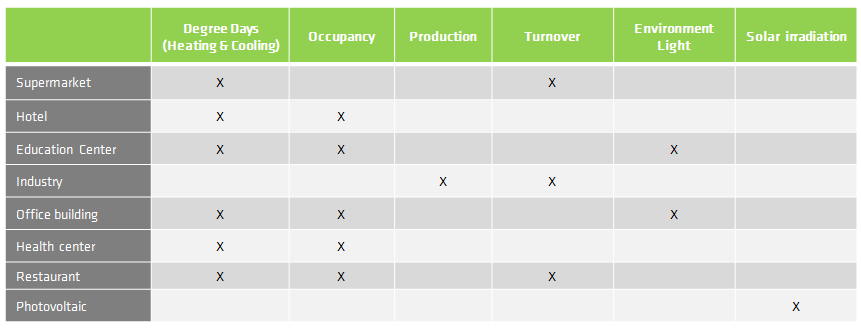Which is the demonstrative period of activity in my facility?

We have to know which is the period of time with a representative cycle of activity in our facility in order to know which is the minimum period of data we should collect before make our baseline. Here, we have some examples: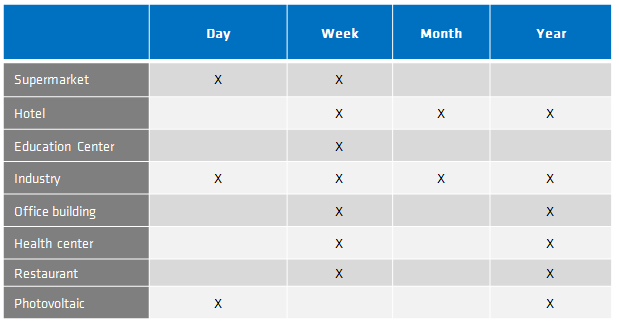Data preprocessing

Once we have determined the variables are going to be correlated with the energetic consumption, we export them of DEXCell Energy Manager and we adequate them for the statistical software we are going to use (EXCEL, Minitab, Stata, Matlab, etc.)

We should remember that there is an interaction between different variables or a variable can be representative if it is squared or cubed. We recommend preparing additional data sets among variables and power calculations.

Moreover, we eliminate those anomalous or unrepresentative data of the installation.

Get the mathematical formula

The mathematical formula is the result of a linear/nonlinear statistical regression. Each of the software procedures performed variously estrus, so we must follow their own tutorials to get the formula.

Each project has its own mathematical formula and there not exist "standard" formulas for a type of installation.

Example of baseline calculation using Minitab 16

Get the statistical error

All statistical process involves a miscalculation. The procedure that we have selected for the calculation of the formula will give a correlation coefficient which will indicate the number of points explained in the  formula

Normally, the indicator is R^ 2.

If we got an R^2 = 98%, this indicates that our formula explains 98% of our consumption, so we will be making an error of 2%.

Enter the adjustment formula in DEXCell EM

Main case

Once the basic project information has been entered, in the reference period tab we can enter our baseline adjustment formula for the theoretical consumption of the project. To do this, we must add those variables that are explanatory in the "Variables" section, define the statistical error in the "Error" section and write the mathematical formula in the "Energy" section: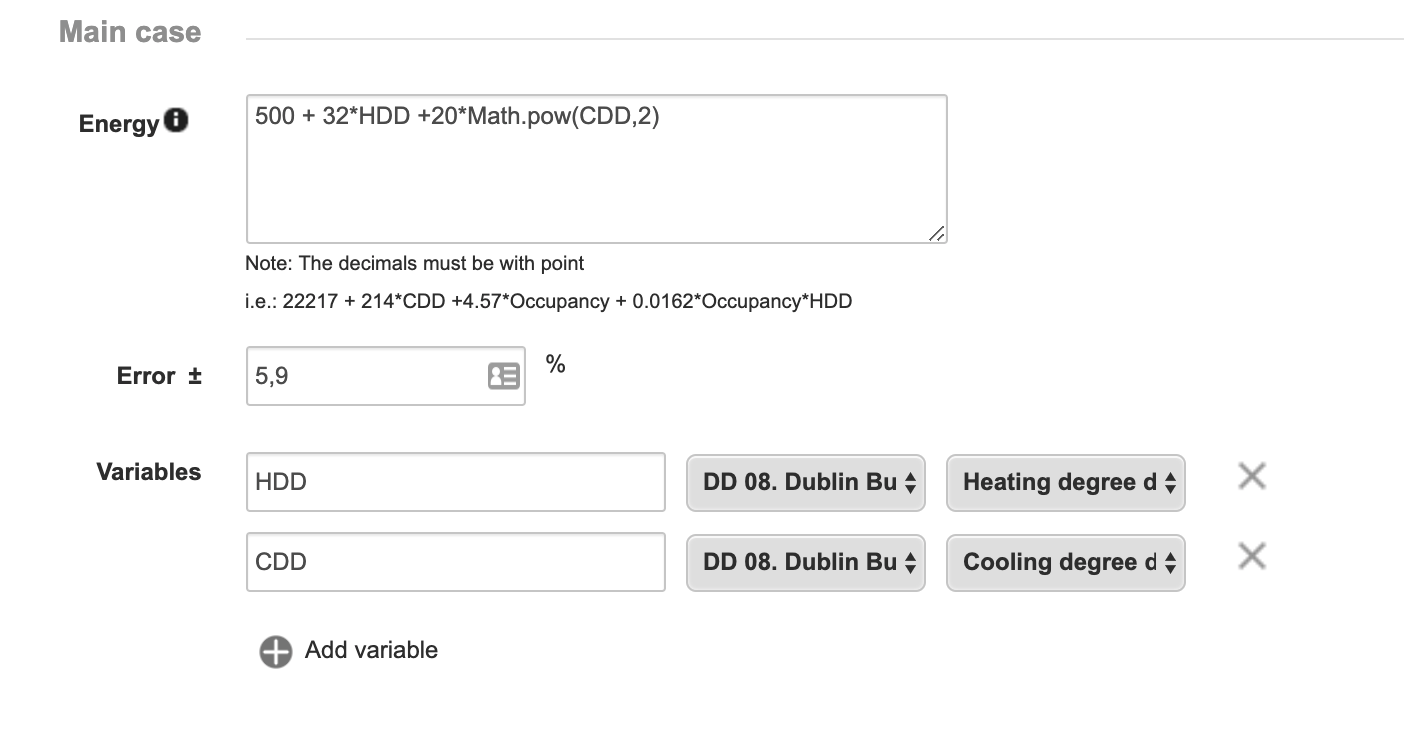For variables you can choose a representative name, and select the device and associated parameter to use them in the formula.

Introducing time conditionals

Maybe, our baseline changes for specific days or periods of time. We can introduce some conditions to change our formula by another one based on the day of a week or months of a year. You can introduce any conditions you need. These will be applied by priority order based on the definition.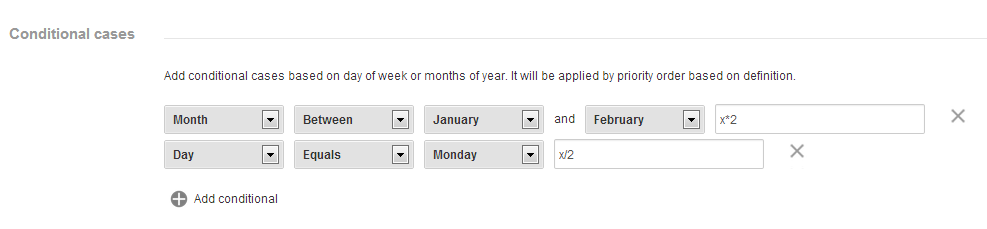The mathematical formulas that can be used are:

 a + b The sum of "a" and "b" a - b The substraction between "a" and "b" a * b The multiplication of "a" and "b" a / b The division of "a" and "b" Math.sqrt(a) Square root of "a" Math.pow(a,b) "a" to the power "b" Math.abs(a) The absolute value of "a" Math.exp(a) Exponent of "a" Math.floor(a) Integer closest to "a", not greater than "a" Math.log(a) Log of "a" base "e" Math.random() Random number 0 to 1 Math.round(a) Integer closest to "a" Math.sin(a) Sine of "a" Math.cos(a) Cosine of "a"

Insert the formula in our Measure & Verification Project

After calculating the formula and the error, we introduce our data in Measure & Verification project and we will have configured our savings for real-time reporting!

Introducing conditionals in the formula

In case we want to introduce a conditional IF in our formula, we should use the following nomenclature:

if (condition1) {

formula1;

} else if (condition2) {

formula2;

} else {

formula3;

}

One example could be by giving different values to energy allocated to heating depending on the temperature:

if (Temp<15) {

4000.3 + 300*Temp;

} else {

2000.8 + 300*Temp;

}

In this case, for temperatures lower than 15ºC the energy would be calculated as E=4000+300*Temperature. For any other case, the energy would be calculated as E=2000+300*Temperature.

3. Generate my baseline based on "reference period"

Calculate the baseline by a "Reference Period" is a powerful option which allows simplifying the calculus of theoretical consumption if we have available data about, at least, one representative activity period of our facility.

When should we use the option of "Reference period"?

-  When we have monitored data, at least, from a representative activity period of my facility.
- When we don't have available external data for correlation (degree day, occupation, etc.) or we don't want to use them.
- When energy  consumption is independent of any external variable measurable
- When the correlation coefficient with external data is very low (less than 20%)

Which is the demonstrative activity period of my facility?

We have to know which is the period of time with a representative cycle of activity in our facility in order to know which is the minimum period of data we should collect before make our baseline. Here, we have some examples:What exactly do the "Temporary Ranges"?

This option aggregates data by mean/ median based on a time window (day, week, month, year) of the amount of data that the client set.

For example, if we are making a substitution of LED's which we have 2 months of data before the date of implementation of the schedules improvements, and our representative period of activity (supermarket) is a week, we can "overlay" 8 weeks with hourly frequency and make the median, obtaining a "approximate" baseline of our theoretical consumption: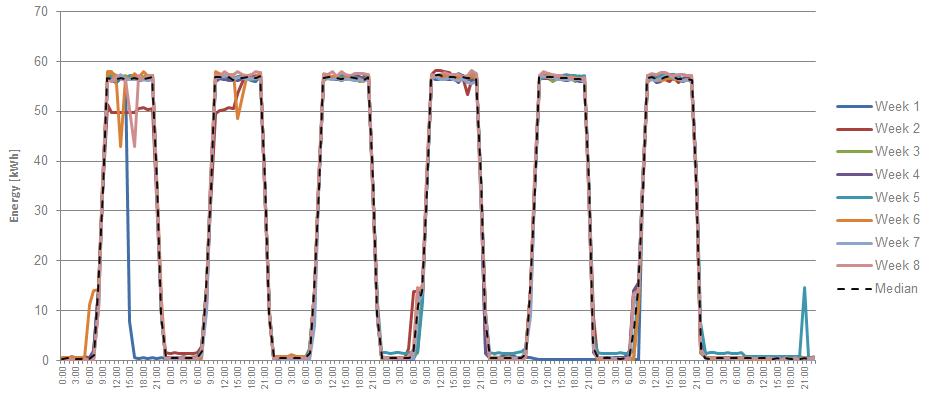How do I set in DEXCell Energy Manager?

1. In step 2 of configuring our "Measure & Verification" project, we select "by temporal ranks"
2. We select the dates where we have data
3. We select the representative time frame for the activity period of our facility (daily, weekly, monthly,...)
4. In the option " Group by" we have to choose between median and average depending on what we think could be better. If our data has a Normal distribution, there won't be any difference. If we have peaks or corrupted data, the median is a more realistic measurement.
5. We can write the error if we know exactly or if we prefer, DEXCell Energy Manager can auto-calculate the error. We just have to click on "Autocalculate error". In the precalculated baseline graph, will be shown with a dashed line the higher and lower margin error in red and green colour.
6. We click on "Preview" and DEXCell Energy Manager will show us the precalculated baseline. If we agree, we click on "Continue" and we follow with the next steps.4. Example of baseline calculation using Minitab

In this section is explained how to calculate the theoretical consumption of a location based on some external parameters.

The location

Activity: Service station, with a coffee shop, shop and restaurant.
Location: Barcelona

Data Requirements

- In the case of a service station, we could discuss if the representative period of activity is a week or a year. Probably the consumption pattern is repeated weekly so with some weeks of data would be enough. On the other hand, if the station has a lot of seasonal climate, it would be convenient to have one year of data.

- As consumption data, we will need, at least, the main consumption of the location

- As external data or consumption variables, it would be ideal to have degree days (CDD, HDD) information and an indicative parameter about the occupation of the service station (tickets or sales).

Available data

Response variable:

• The main consumption of the installation from January 2012 to December 2012 (1 year), hourly frequency

Explicative variables:

• Heating and cooling degree days (obtained from degreedays.net) (HDD, CDD)

• Daily sales (S)

• Daily tickets (T)

• Interaction between variables

• It is interesting to generate new data sets transforming existing variables and study if correlated with consumption. For this example, we will create square and cubic variables of the tickets (T) and Sales (S) and the product of Sales*Heating degree days and Sales*Cooling degree days.

• HDD². HDD³

• CDD², CDD³

• T², S²

• S*HDD, S*CDD

Resolution or baseline frequency

The resolution or baseline resolution is affected by the resolution of the available variables. In our case, Degree Days, tickets and Sales has "Daily" resolution, so this will be the resolution of our formula.

Calculating the formula with Minitab 16

Minitab 16 is a statistical software useful for this type of problems. There exists more software which can help us, but Minitab 16 is one of the most used in the field of engineering statistics.

Once we have installed, we open and we introduce our data in columns as we can see in the following figure: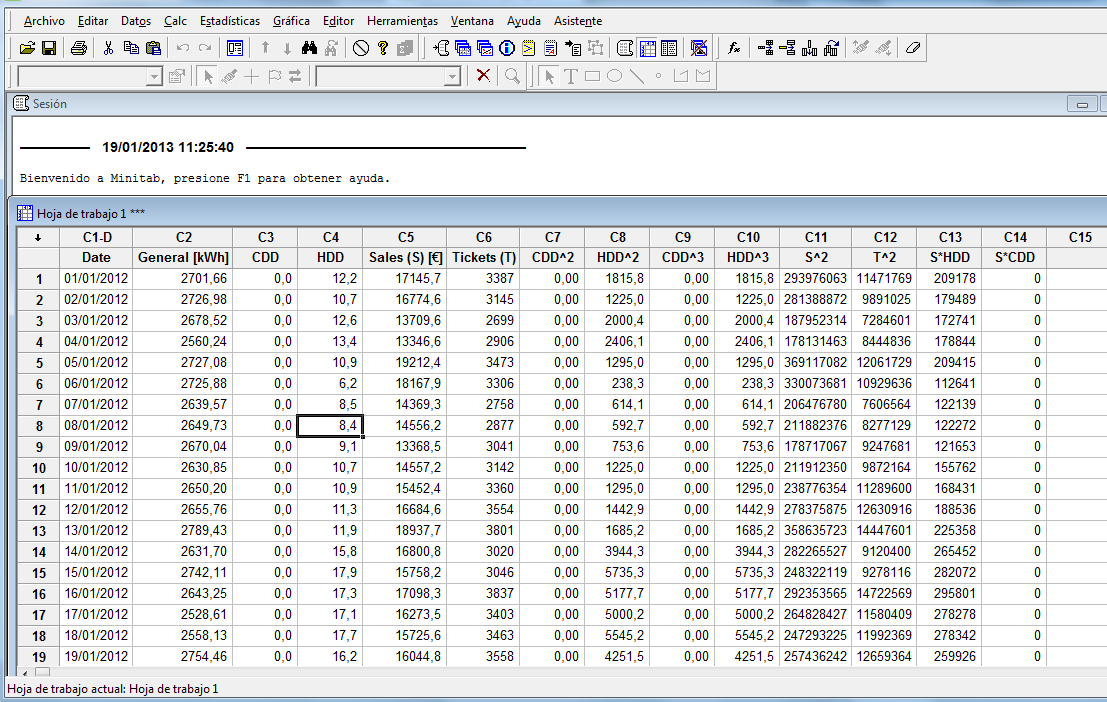Note: In the image above there is an error with the first HDD^2 values, as they are HDD^3.

The tool we are going to use in order to calculate the formula is called "Regression". If we want an exhaustive analysis where we need more accuracy, we can use "Regression step by step".

We click in "Statistics" > "Regression" > "Regression..."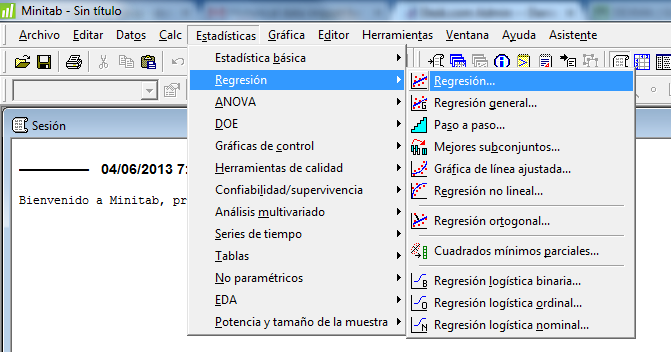As Response we select "Main [kWh]" and as a "Predictors" the rest of variables expect the "Date", because it's not necessary for calculate the formula. The variables are selected by double-clicking on them..

Note: If you are interested in observing the residual values in graphical format, in "Graphics" you can activate the "four in one" option. See residues (error) in a graphical format will allow detecting outliers which can be eliminated from the analysis.

We click on "Accept" and Minitab will calculate automatically the equation based on the predictors selected, the correlation coefficient (R-cuad). Then, we have to refine the process.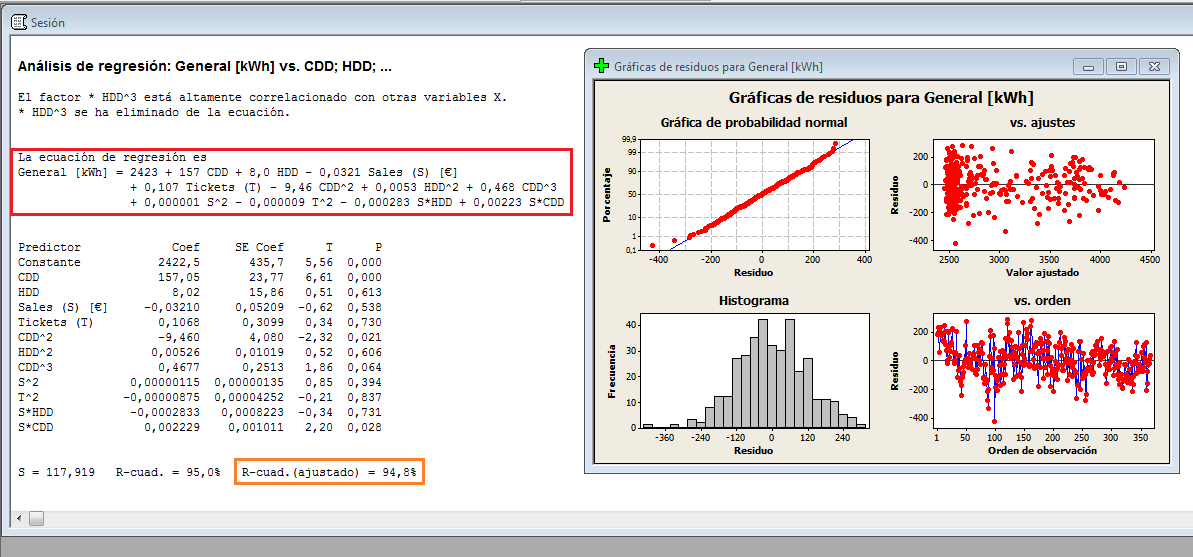Refining the equation - statistical P value

The first equation estimated is not necessarily the best. In fact, we have introduced in the model a set of interactions between variables and transformations that maybe don't correlate with the model.

One way to understand if a variable correlated or not is to look at the P value.  If it's greater than 0.05 indicates that the variable in the model does not correlate. We can see that our first model has up to 7 variables that do not correlate: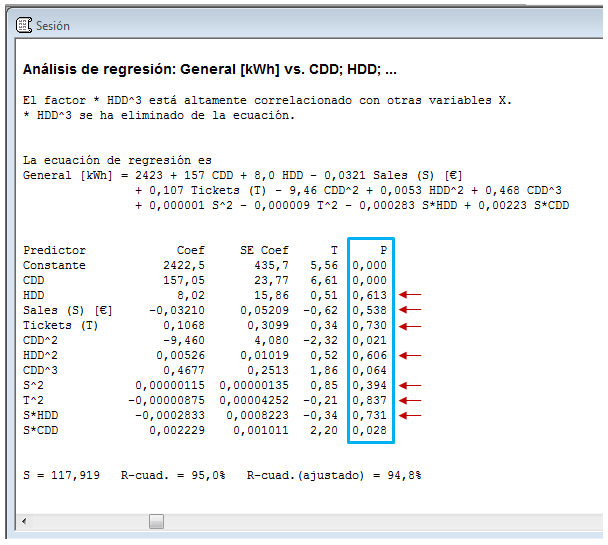We don't have to eliminate all the variables which not correlate because there they are related between them. We have to remove one by one, from highest to lowest P value and analyse iteration by iteration what values of P we receive.

Moreover, it may happen that after removing one of the variables, if we introduce it again, we will give a P value less than 0.05.

Therefore, the refinement process it's not concrete, and can exists infinite combinations. We must decide when the model is correct for our purpose and the iterations.

One of our results could be this one: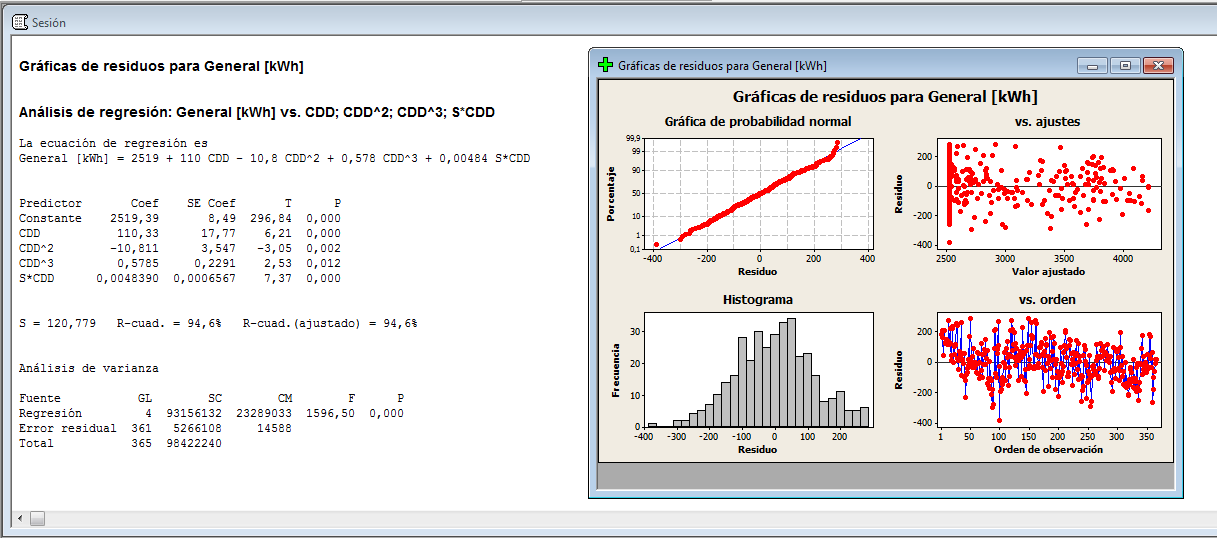We can see that the formula has been simplified, using only Cooling degree days (CDD) and sales (S) as predictors. We see how the formula is a nonlinear grade 3 polynomial. We have the cubic CDD variable and the interaction of CDD with Sales (S), indicating more heating is related to more sales.

The correlation value is so good (94.4%), which indicates an error in our savings about a 5.6%

Outliers

With the residual chart, we will observe if exists some outliers in the model or not, indicating a lower correlation coefficient and a bigger error. If so, you can reach an agreement with the client to delete this data from the model. Sometimes it can happen that up to 30% of the data received are outliers and has to be removed.

Once we have calculated our formula, it's time to insert it to our Measure & Verification project in DEXCell Energy Manager!

Generate my baseline based on a formula

1 out of 1 found this helpful